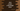# Create a Rectangle class in Java and calculate area, perimeter## How to create a Rectangle class in Java and calculate area and perimeter:

In this post, we will learn how to create a Rectangle class in Java and how to calculate the area and perimeter. With this tutorial, you will learn how to create a class in Java and how to access its methods and parameters.

### Steps for the program:

We will follow the following steps:

1. Create one Rectangle class.
2. This class will take the height and width of the rectangle in its constructor.
3. This class will hold two more methods to calculate the area and perimeter of the rectangle by using the provided health and width values.
4. We will use (width * height) to find the area and 2 * (width + height) to find the perimeter.

### Java program:

Let’s take a look at the Java program:

We will create two classes. One is the main class and another is a class to hold the rectangle data.

### Rectangle.java:

``````public class Rectangle {
double w, h;

Rectangle(double width, double height) {
this.w = width;
this.h = height;
}

public double getArea() {
return w * h;
}

public double getPerimeter() {
return 2 * (w + h);
}
}``````

This class is to hold the width and height values of the rectangle. It has one constructor that takes the width and height and assigns them to the local variables w and h.

getArea method return the area for the saved width and height.

getPerimeter method return the perimeter.

### Main.java:

This is the main file. It takes user input values for the width and height and calls the Rectangle class.

``````import java.util.Scanner;

class Main {
public static void main(String[] args) {
double width, height;

Scanner sc = new Scanner(System.in);

System.out.println("Enter the width of the rectangle: ");
width = sc.nextDouble();

System.out.println("Enter the height of the rectangle: ");
height = sc.nextDouble();

Rectangle rectangle = new Rectangle(width, height);

System.out.println("Area: "+rectangle.getArea());
System.out.println("Perimeter: "+rectangle.getPerimeter());
}
}``````
• It takes the width and height as inputs from the user and stores in the variables width and height.
• sc is a Scanner object used to read user inputs.
• It creates one Rectangle object by using the user input width and height values.
• The last two lines are printing the area and perimeter for the rectangle. It calls getArea and getPerimeter methods of this object.

### Sample output:

If you run this program, it will give output as like below:

``````Enter the width of the rectangle:
10
Enter the height of the rectangle:
12
Area: 120.0
Perimeter: 44.0``````

We can also create other methods as well in the Rectangle class to calculate different other things that are related to a rectangle.